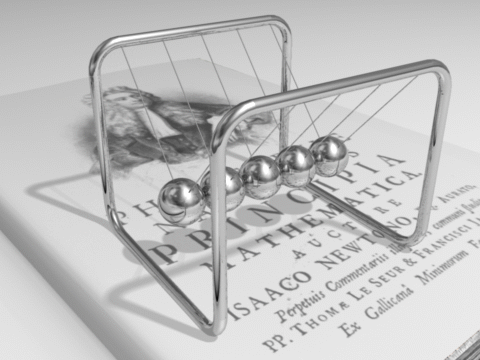$$\require{cancel}$$

7.2: Conservation of Momentum

Internal vs. External Forces

Net external forces (that are nonzero) change the total momentum of the system, while internal forces do not.

learning objectives

• Contrast the effects of external and internal forces on linear momentum and collisions

Linear Momentum and Collisions

Newton’s 2nd law, applied to an isolated system composed of particles, $$\mathrm{F_{tot}=\frac{dp_{tot}}{dt}=0}$$ indicates that the total momentum of the entire system $$\mathrm{p_{tot}}$$ should be constant in the absence of net external forces. Forces external to the system may change the total momentum when their sum is not 0, but internal forces, regardless of the nature of the forces, will not contribute to the change in the total momentum. To analyze a mechanical system, it is important to recognize which forces are internal and which are external. Once a mechanical system is clearly defined, it’s not hard to understand what part should be considered external.Newton’s Cradle: Total momentum of the system (or Cradle) is conserved. (neglecting frictional loss in the system. )

• External forces: forces caused by external agent outside of the system.
• Internal forces: forces exchanged by the particles in the system.

To give you a better idea, let’s consider a simple example. We have two hockey pucks sliding across a frictionless surface, and we neglect air resistance for simplicity. They collide with each other at t=0.

Let’s first list all the forces present in the system. There are mainly three kinds of forces: Gravity, normal force (between ice & pucks), and frictional forces during the collision between the pucks

How should we define our system? In most cases, we would be interested in the motion of the pucks (and nothing else). Therefore, our system consists of two pucks (and nothing else). All the rest of the universe becomes external. With this in mind, we can see that gravity and normal forces are external, while the frictional forces between pucks are internal. Since all the external forces cancel out with each other, there are no net external forces. (Gravity and normal force on each puck have the same magnitude, but are in the opposite directions) Therefore, we conclude that the total momentum of the two pucks should be a conserved quantity.

• In the previous example, it is worthwhile to note that we didn’t assume anything about the nature of the collision between the two pucks. Without knowing anything about the internal forces (frictional forces during contact), we learned that the total momentum of the system is a conserved quantity (p1 and p2 are momentum vectors of the pucks. ) In fact, this relation holds true both in elastic or inelastic collisions. Whether the total kinetic energy of the pucks is conserved or not, total momentum is conserved.
• Also note that, in the previous example, if we include the rest of the Earth in our system, the gravity and normal forces themselves become internal.

Key Points

• External forces are forces caused by external agent outside of the system.
• Internal forces are forces exchanged by the objects in the system.
• To determine what part should be considered external and internal, mechanical system should be clearly defined.

Key Terms

• inelastic: (As referring to an inelastic collision, in contrast to an elastic collision. ) A collision in which kinetic energy is not conserved.
• elastic: referring to elastic collision, in contrast to inelastic collision. A collision in which kinetic energy is conserved